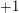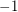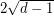# eigenvalue

## Signing a graph to have small magnitude eigenvalues ★★

Author(s): Bilu; Linial

Conjecture   Ifis the adjacency matrix of a-regular graph, then there is a symmetric signing of(i.e. replace someentries by) so that the resulting matrix has all eigenvalues of magnitude at most.

Keywords: eigenvalue; expander; Ramanujan graph; signed graph; signing